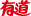有道词典

[数] partial correlation

• 1. 偏相关: Partial correlation | Partial | PAC | net correlation
• 2. 非线性偏长相关: nonlinear irreducible long term dependence
• 3. 非线性偏自相关: nonlinear partial autocorrelation

• 1.本文用偏相关系数计算方法，通过归一化处理等得到一组独立性权数。
In this paper the partial correlation coefficients may be used to calculate the weight of independence.
dict.cnki.net
• 2.运用偏相关分析和主成分回归的方法，研究它们与地理因素的关系。
Their relation with geographical factors was studied by partial correlation analysis in combination with regression of principal components.
dict.cnki.net
• 3.本文论述多元线性回归中的回归系数与偏相关系数之间的数量关系以及它们的统计意义。
This paper deals with the quantitative relations between regression coefficients and partial correlation coefficients in multivariate linear regression, and their statistical meanings.
dict.youdao.com